# Capacitance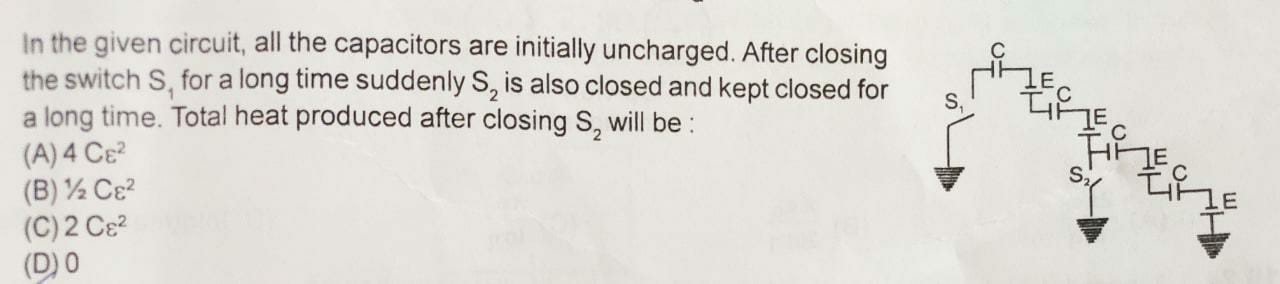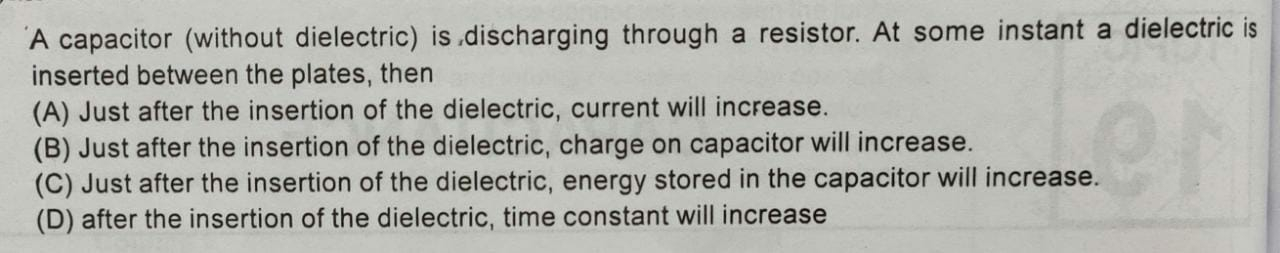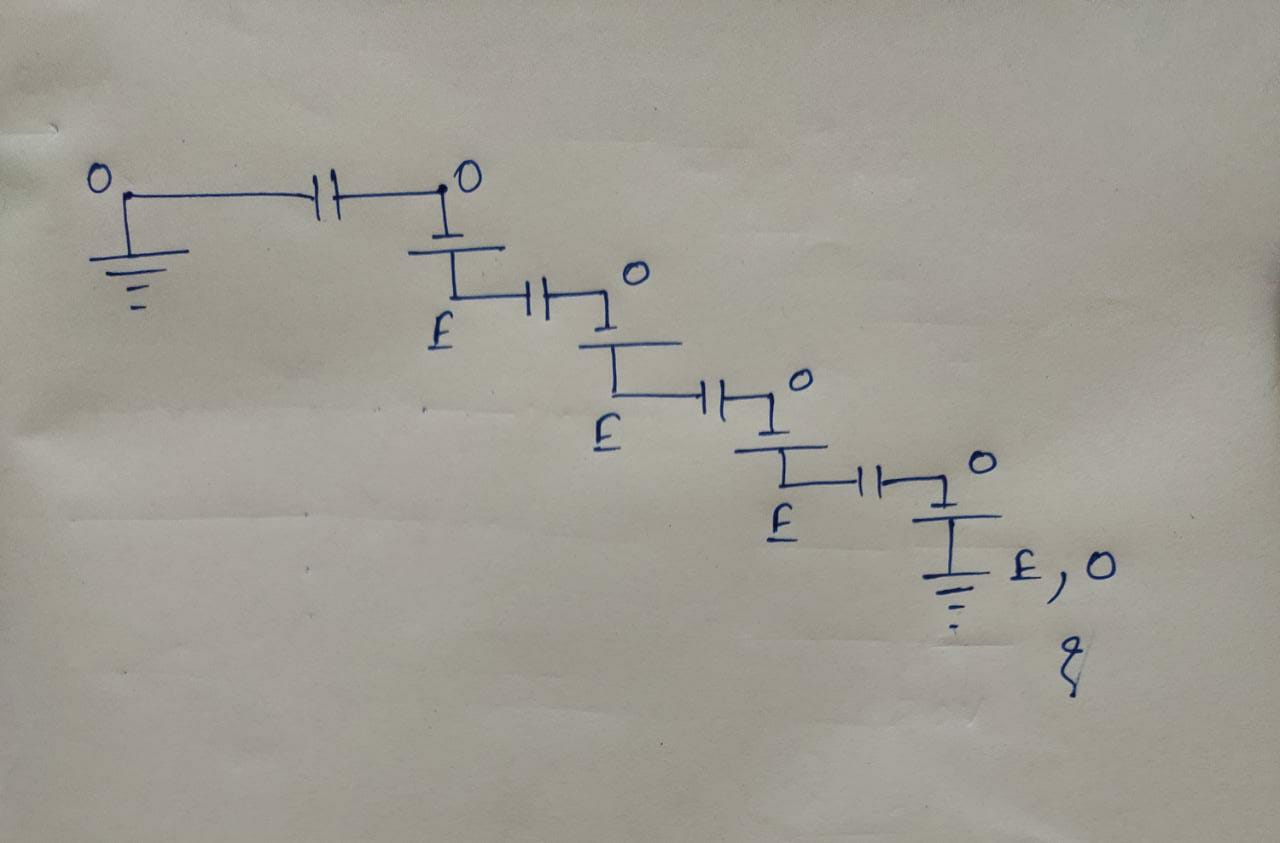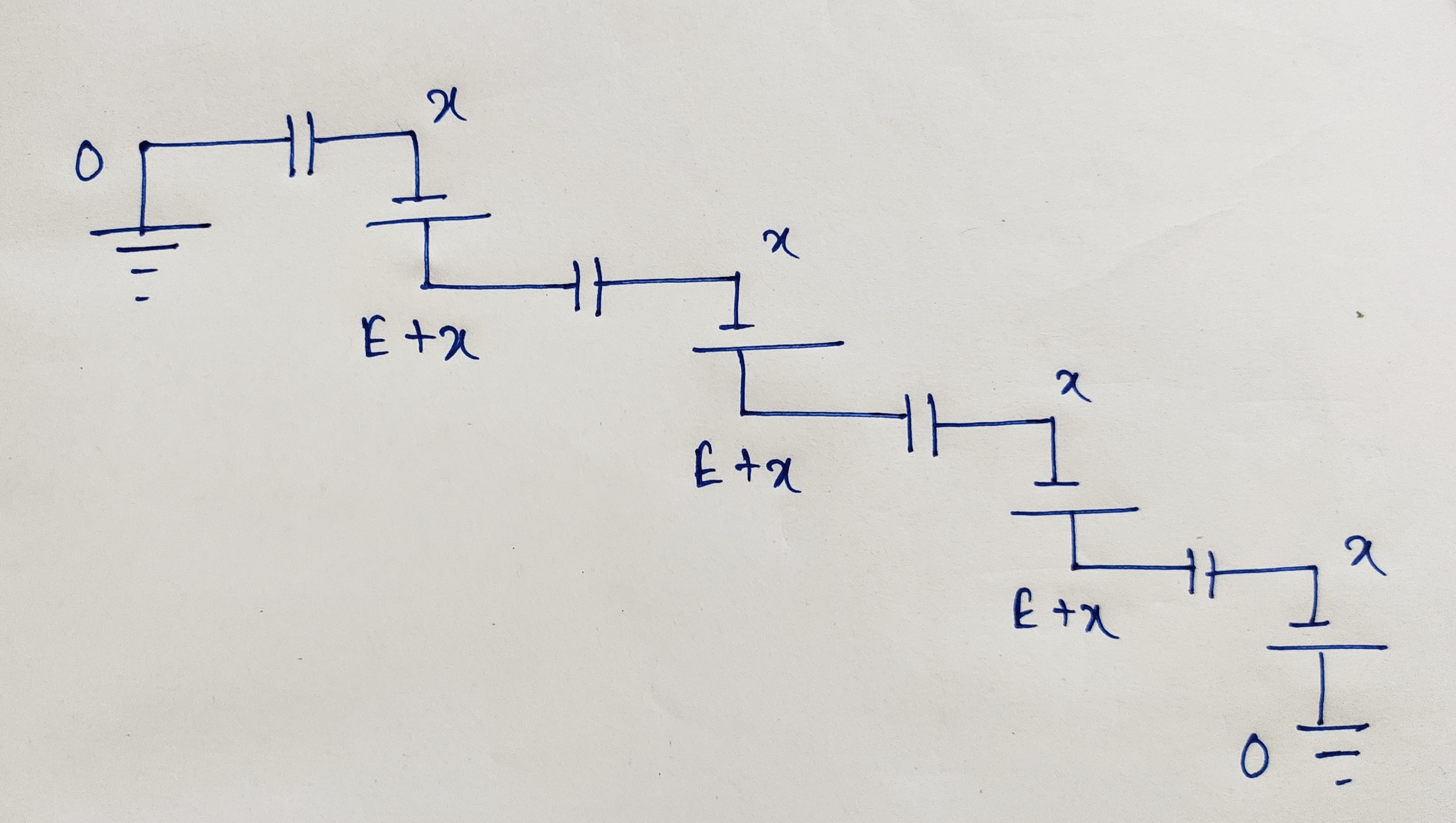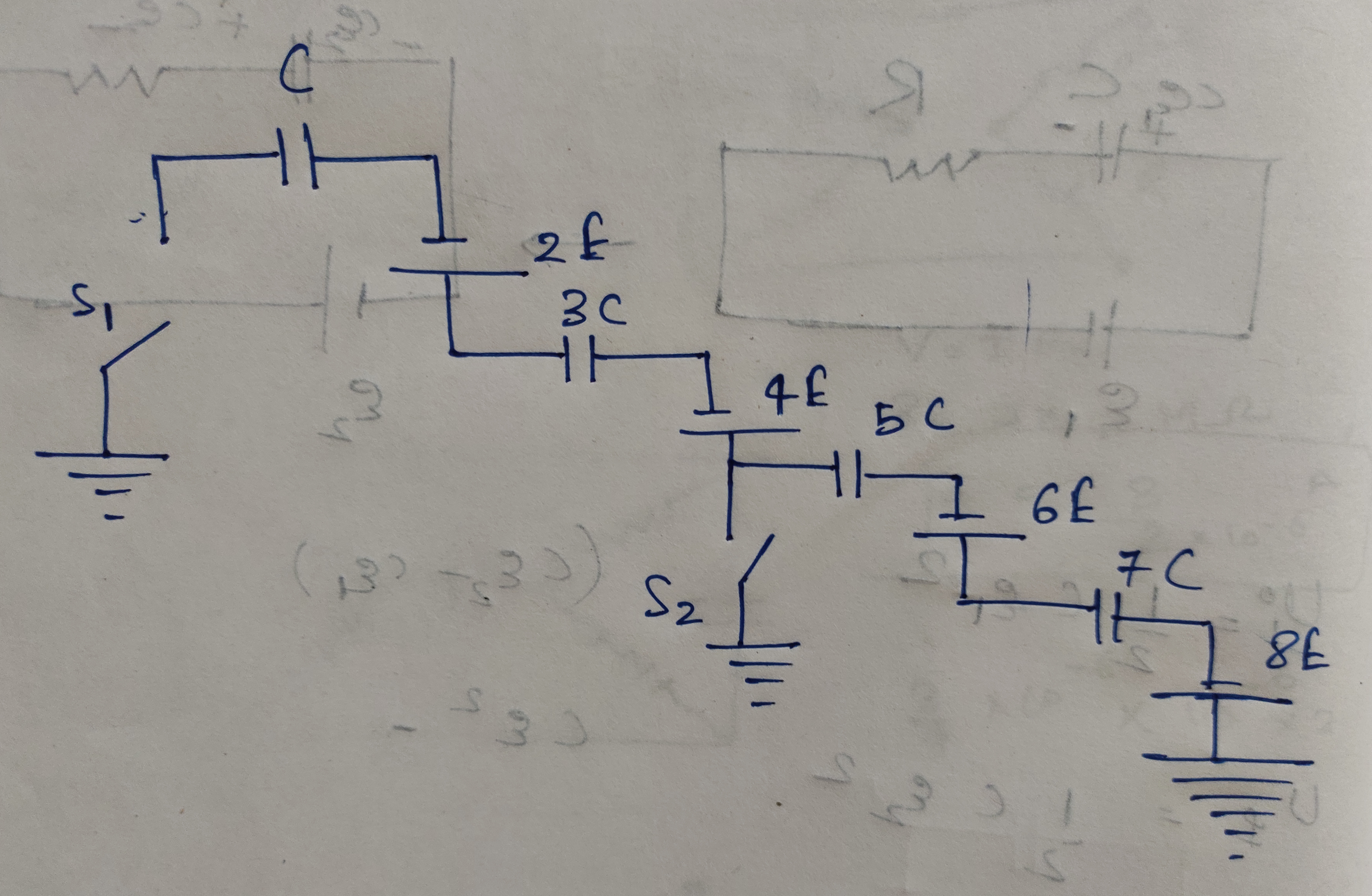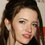Note by Talulah Riley
8 months, 1 week ago

This discussion board is a place to discuss our Daily Challenges and the math and science related to those challenges. Explanations are more than just a solution — they should explain the steps and thinking strategies that you used to obtain the solution. Comments should further the discussion of math and science.

When posting on Brilliant:

• Use the emojis to react to an explanation, whether you're congratulating a job well done , or just really confused .
• Ask specific questions about the challenge or the steps in somebody's explanation. Well-posed questions can add a lot to the discussion, but posting "I don't understand!" doesn't help anyone.
• Try to contribute something new to the discussion, whether it is an extension, generalization or other idea related to the challenge.
• Stay on topic — we're all here to learn more about math and science, not to hear about your favorite get-rich-quick scheme or current world events.

MarkdownAppears as
*italics* or _italics_ italics
**bold** or __bold__ bold
- bulleted- list
• bulleted
• list
1. numbered2. list
1. numbered
2. list
Note: you must add a full line of space before and after lists for them to show up correctly
paragraph 1paragraph 2

paragraph 1

paragraph 2

[example link](https://brilliant.org)example link
> This is a quote
This is a quote
    # I indented these lines
# 4 spaces, and now they show
# up as a code block.

print "hello world"
# I indented these lines
# 4 spaces, and now they show
# up as a code block.

print "hello world"
MathAppears as
Remember to wrap math in $$ ... $$ or $ ... $ to ensure proper formatting.
2 \times 3 $2 \times 3$
2^{34} $2^{34}$
a_{i-1} $a_{i-1}$
\frac{2}{3} $\frac{2}{3}$
\sqrt{2} $\sqrt{2}$
\sum_{i=1}^3 $\sum_{i=1}^3$
\sin \theta $\sin \theta$
\boxed{123} $\boxed{123}$

## Comments

Sort by:

Top Newest

There are two possibilities:

1) The capacitor charge stays the same
2) The capacitor energy stays the same

It is not entirely clear to me which possibility is more valid. I will assume that the first is true. The consequences are:

1) The capacitor voltage decreases, so the current in the circuit decreases
2) The energy stored in the capacitor decreases
3) The capacitance increases, so the time constant $\tau = R C$ increases

Answer $D$ is true no matter which assumption we initially make. But the assumption of constant charge makes $D$ the only correct answer. See the code below for some trials and results.

  1 2 3 4 5 6 7 8 9 10 11 12 13 14 15 16 17 18 19 20 21 22 23 24 25 26 27 28 29 30 31 32 33 34 35 36 37 38 39 40 41 42 43 44 45 46 47 48 49 50 51 52 53 import math # General principles # Q = C*V # E = 0.5*C*(V**2.0) # C is proportional to epsilon (dielectric strength) # dielectric has permittivity greater than that of air # Assumptions for when dielectric inserted: # 1) Charge stays the same # 2) Capacitance increases ########################################### # Case 1 Q1 = 2.0 C1 = 3.0 V1 = Q1/C1 E1 = 0.5*C1*(V1**2.0) print V1 print E1 print "" print "" ########################################### # Case 2 Q2 = 2.0 # same as in Case 1 C2 = 5.0 # greater than in Case 1 V2 = Q2/C2 E2 = 0.5*C2*(V2**2.0) print V2 print E2 ########################################### # Results #>>> #0.666666666667 #0.666666666667 #0.4 #0.4 #>>> 

- 8 months, 1 week ago

Log in to reply

@Steven Chase yes D is the correct answer. Thanks for the solution.

- 8 months, 1 week ago

Log in to reply

@Steven Chase @Karan Chatrath Thanks in advance

- 8 months, 1 week ago

Log in to reply

@Steven Chase I have uploaded a new problem above in this note only. Have a look. Thanks in advance

- 8 months, 1 week ago

Log in to reply

With just S1 closed, there are 4 sources and 4 capacitors in series. So initially, each capacitor has voltage E. After closing S2, there are now two separate circuits, each with 2 sources and 2 capacitors in series. Since the ratio of sources to capacitors in each circuit is the same as in the initial case, the capacitor voltages remain the same, and no heat is dissipated.

- 8 months, 1 week ago

Log in to reply

@Steven Chase in have doubt . Let me share my rough work .

- 8 months, 1 week ago

Log in to reply

@Steven Chase I have attached my rough work above. I am having great confusion. If we see clearly the potential difference across first capacitor is not E?

- 8 months, 1 week ago

Log in to reply

The non-polarity side of a source is not at zero volts. It is at a potential E volts less than the polarity side. The ground points are all at zero volts.

- 8 months, 1 week ago

Log in to reply

@Steven Chase But why E volts less. A battery ensures that it should hai potential differnce of E accross it. So why my work is incorrect?

- 8 months, 1 week ago

Log in to reply

The potential at the polarity side of the battery is E volts greater than the potential at the non-polarity side. That does not mean that the voltage at the non-polarity side is "zero". In general, you can choose any single place in the circuit you want to be zero, and then you must be consistent from then on. All the ground (earth) points are at the same potential. By convention, I am saying that those points are at zero potential.

- 8 months, 1 week ago

Log in to reply

@Steven Chase Ohkay i have again added my rough work. Is it correct now.?

- 8 months, 1 week ago

Log in to reply

Yes, and $x = - E$

- 8 months, 1 week ago

Log in to reply

@Steven Chase how do you know $x=-E$????

- 8 months, 1 week ago

Log in to reply

All the capacitor voltages are the same, since they are in series

- 8 months, 1 week ago

Log in to reply

@Steven Chase Thanks for clearing doubts. I have added a new figure with the same problem.
Now, I am. Very interested to see how you will approach the problem.

- 8 months, 1 week ago

Log in to reply

×

Problem Loading...

Note Loading...

Set Loading...